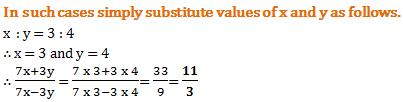# Aptitude Test for Computer and IT Engineers - Set 3

1)   5% of 5% of Rs. 100 is

a. Rs. 0.25
b. Rs. 0.50
c. Rs. 10
d. Rs. 25
 Answer  Explanation ANSWER: Rs. 0.25 Explanation: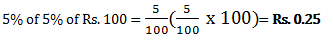2)   If 20% of an electricity bill is deducted, then Rs. 100 is still to be paid. How much was the original bill?

a. Rs. 110
b. Rs. 115
c. Rs. 120
d. Rs. 125
 Answer  Explanation ANSWER: Rs. 125 Explanation: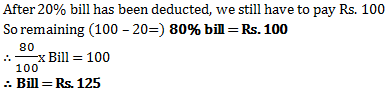3)   If X% of Y is 100 and Y% of Z is 200, find a relation between X and Z.

a. Z = X/2
b. Z = 2X
c. X = Z/4
d. Z = 4X
 Answer  Explanation ANSWER: Z = 2X Explanation: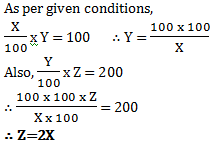4)   40% of greater number is equal to 60% of the smaller. If their sum is 150, then the greater number is

a. 70
b. 80
c. 90
d. 60
 Answer  Explanation ANSWER: 90 Explanation: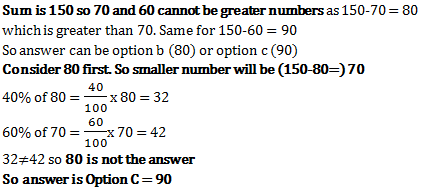5)   Two numbers are less than a third number by 30% and 37% respectively. How much percent is the second number less than the first?

a. 7%
b. 10%
c. 4%
d. 3%
 Answer  Explanation ANSWER: 10% Explanation: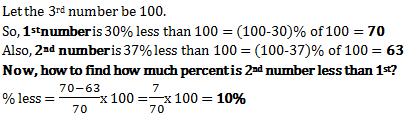6)   The difference between simple and compound interest on a certain sum of money for 2 years at 4 percent per annum is Re.1. The sum of money is

a. Rs. 600
b. Rs. 625
c. Rs. 560
d. Rs. 650
 Answer  Explanation ANSWER: Rs. 625 Explanation: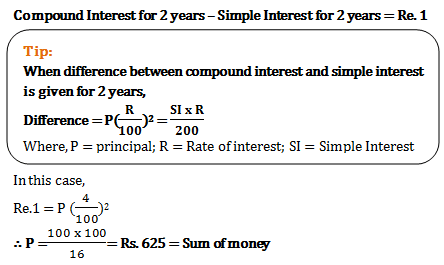7)   A sum of money invested at compound interest amounts to Rs. 800 in 3 years and to Rs. 840 in 4 years. The rate of interest per annum is

a. 2(1/2)%
b. 4%
c. 5%
d. 6(2/3)%
 Answer  Explanation ANSWER: 5% Explanation: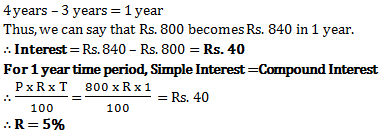8)   If simple interest on a certain sum for 15 months at 7(1/2) % per annum exceeds the simple interest on the same sum for 8 months at 12(1/2)% per annum by Rs. 32.50, then the sum (in Rs.) is

a. 312
b. 312.50
c. 3120
d. 3120.50
 Answer  Explanation ANSWER: 3120 Explanation: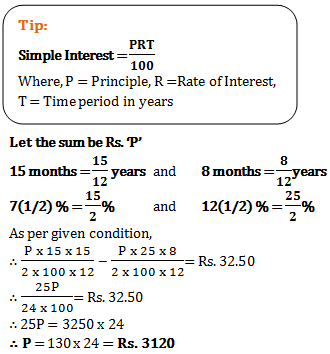9)   In 4 years the simple interest on certain sum of money is 9/25 of the principal. The annual rate of interest is

a. 4%
b. 4(1/2)%
c. 9%
d. 10%
 Answer  Explanation ANSWER: 9% Explanation: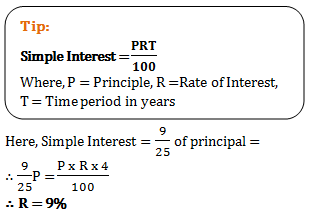10)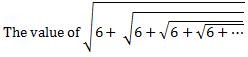a. 2
b. 5
c. 4
d. 3
 Answer  Explanation ANSWER: 3 Explanation: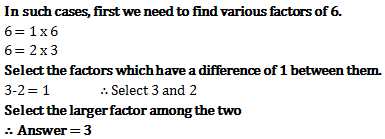11)   Which is greatest among the following numbers?
22 , 7 , 23 , 5

a. 7
b. 22
c. 23
d. 5
 Answer  Explanation ANSWER: 23 Explanation: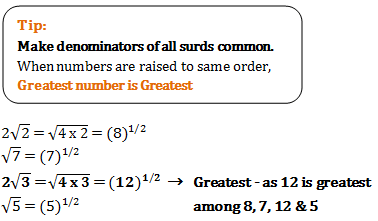12)   Simplify:
 0.72 x 0.72 x 0.72 - 0.39 x 0.39 x 0.39 0.72 x 0.72 + 0.72 x 0.39 + 0.39 x 0.39

a. 0.333
b. 0.33
c. 0.3333
d. 0.3334
 Answer  Explanation ANSWER: 0.33 Explanation: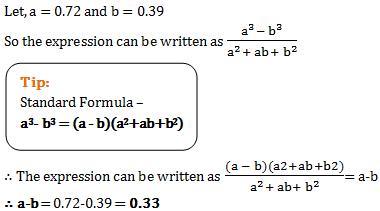13)   The product of two positive numbers is 4752 and their ratio is 11:12. The smaller of these numbers is

a. 72
b. 60
c. 66
d. 75
 Answer  Explanation ANSWER: 66 Explanation: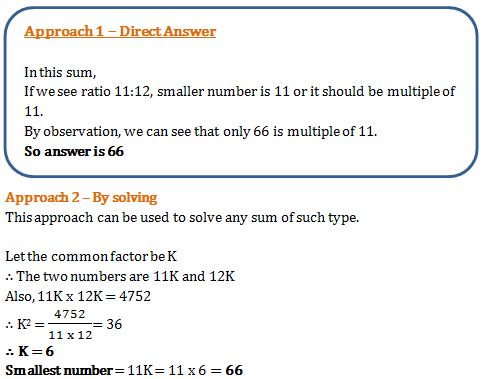14)   Sum of squares of two numbers is 145. If square root of one number is 3, find the other number.

a. 136
b. 9
c. 64
d. 8
 Answer  Explanation ANSWER: 8 Explanation: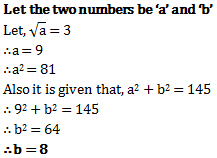15)   If a person sells a ‘sari’ for Rs. 5200, making a profit of 30%, then the cost price of the sari is

a. Rs. 4420
b. Rs. 4000
c. Rs. 3900
d. Rs. 3800
 Answer  Explanation ANSWER: Rs. 4000 Explanation: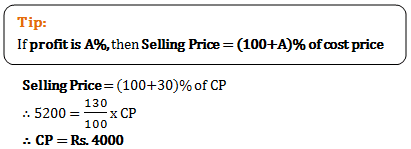16)   The ratio of cost price and selling price is 4:5. The profit percent is

a. 10%
b. 20%
c. 25%
d. 30%
 Answer  Explanation ANSWER: 25% Explanation: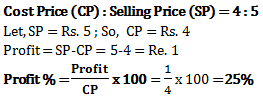17)   Two bicycles were sold for Rs. 3990 each, gaining 5% on one and losing 5% on the other. The gain or loss percent on the whole transaction is

a. Neither gain nor loss
b. 2.5% gain
c. 2.5% loss
d. 0.25% loss
 Answer  Explanation ANSWER: 0.25% loss Explanation: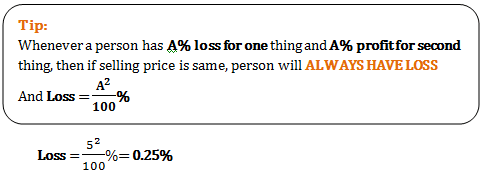18)   If selling price of 40 articles is equal to cost price of 50 articles, the loss or gain percent is

a. 25% loss
b. 20% loss
c. 25% gain
d. 20% gain
 Answer  Explanation ANSWER: 25% gain Explanation: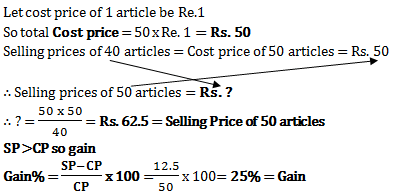19)   If x:y = 3:4, then (7x+3y):(7x-3y) is equal to

a. 5:2
b. 4:3
c. 11:3
d. 37:19
 Answer  Explanation ANSWER: 11:3 Explanation: